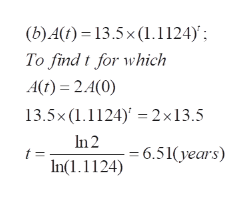# In 1980, direct U.S. business investment abroad was about 13.5 billion dollars. From 1980 through 2010, that investment grew at an average annual rate of 11.24%.†(a)Make an exponential model that shows the U.S. direct investment abroad A, in billions of dollars, t years after 1980.A(t) =    (b)From 1980, how long did it take (in years) for U.S. investments abroad to double? (Round your answer to two decimal places.) years(c)According to the model, how long would it take (in years) from 2010 for investments abroad to double the level present in 2010? (Round your answer to two decimal places.) years

Question
167 views
In 1980, direct U.S. business investment abroad was about 13.5 billion dollars. From 1980 through 2010, that investment grew at an average annual rate of 11.24%.†
(a)
Make an exponential model that shows the U.S. direct investment abroad A, in billions of dollars, t years after 1980.
A(t) =

(b)
From 1980, how long did it take (in years) for U.S. investments abroad to double? (Round your answer to two decimal places.)
years
(c)
According to the model, how long would it take (in years) from 2010 for investments abroad to double the level present in 2010? (Round your answer to two decimal places.)
years
check_circle

Step 1

To determine the exponential model corresponding to the given data

Step 2

(a) In an exponential model , the variable t occurs as an exponent. In this problem, A(t+1) is 1.1124 times A(t). Hence the  model is given by the equation , as shown.

Step 3

(b) We require t such that A(t)...help_outlineImage Transcriptionclose(b) A(t) = 13.5 x (1. 1124)'; To find t for which A) 2.4(0) 13.5x (1.1124 2x13.5 In 2 =6.51(years) n(1.1124) fullscreen

### Want to see the full answer?

See Solution

#### Want to see this answer and more?

Solutions are written by subject experts who are available 24/7. Questions are typically answered within 1 hour.*

See Solution
*Response times may vary by subject and question.
Tagged in

### Math